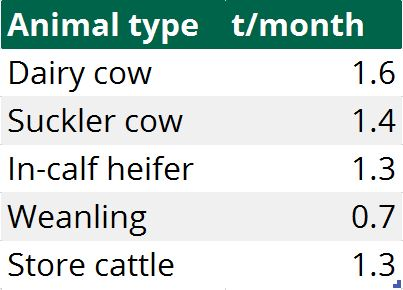With the recent burst of growth and silage harvesting, many farmers are asking themselves if they have enough silage in the yard to get them through another winter.

To figure this out, it’s important to know exactly how much silage you have in your pit; how many head of stock you will have on your farm over the winter; and what exactly each animal will eat.

Teagasc has developed a simple guide to help you see if you have enough silage in your yard for the coming winter.

Calculating how much silage is in your pit:
• Step 1: Measure length, width and height of pit in metres;
• Step 2: Multiply length x width x height = total silage area available;
• Step 3: Estimate the dry matter (DM) of your silage;
• Step 4: Multiply the total area by either 0.68, 0.77 or 0.81 (depending on silage DM);
• Step 5: Calculate how long the silage will last for the animals on your farm.

If the silage is 18% DM, multiply the area by 0.81. If it’s 20% DM, multiply by 0.77 or if your silage has a DM content of 25% you will need to multiply the area by 0.68 to calculate how much silage is actually in your pit.

## Example: How much is in the pit?

Pit height (2m) x pit width (10m) x pit length (20m) = area (400m³).

If the silage has an estimated DM of 25%, the amount of silage available = 400 x 0.68 = 272t fresh weight (FW).

## How long will the silage last?

How long the silage will last depends on the type of animals present on your farm and how much of the feedstuff they will eat over the winter months.

For example, a dairy cow will eat 1.6t/month and a weanling will eat 1.3t/month. If we have 50 dairy cows and 40 weanlings, the calculation would be (50 x 1.6) + (40 x 1.3) = 132t/month requirement.

Divide the total available silage by the monthly requirement to calculate how many months of feed you have available.

Example:

272 divided by 132 = 2.06 months’ worth of silage.Source: Teagasc

In this case, the farmer probably won’t have enough silage and should consider buying more or making a second cut.

## Baled silage

According to Teagasc, the weight of a round bale of silage is 200-240kg DM. At a DM feeding rate of 4kg/day, this would supply 50 cows with enough feed for one day. Bales shouldn’t be forgotten about when calculating how much silage you have available.